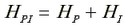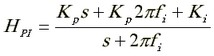﻿ 20-sim webhelp > Toolboxes > Control Toolbox > Filter Editor > Controllers > PI-Controller

# PI-Controller

The PI Controller consists of an integrator with a proportional gain.

 • Gain Kp: The controller gain Kp determines the high frequency gain of the controller.
 • Gain Ki: The controller gain Ki determines the frequency gain of the controller at frequency w = 1 [rad/s].
 • Frequency fi: The integration behavior of the controller stops after frequency fi in Hz. A decrease of this frequency corresponds to a more pure integration behavior.

## Transfer function of the controller:that equals